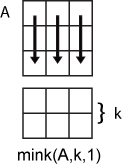# mink

Find `k` smallest elements of array

## Syntax

``B = mink(A,k)``
``B = mink(A,k,dim)``
``B = mink(___,'ComparisonMethod',c)``
``[B,I] = mink(___)``

## Description

example

````B = mink(A,k)` returns the `k` smallest elements of `A`. If `A` is a vector, then `mink` returns a vector containing the `k` smallest elements of `A`.If `A` is a matrix, then `mink` returns a matrix whose columns contain the `k` smallest elements of each column of `A`.If `A` is a multidimensional array, then `mink` returns the `k` smallest elements along the first dimension whose size does not equal 1. ```

example

````B = mink(A,k,dim)` determines the `k` smallest elements of `A` along dimension `dim`.```

example

````B = mink(___,'ComparisonMethod',c)` optionally specifies how to compare elements of `A` for any of the previous syntaxes. For example, `mink(A,k,'ComparisonMethod','abs')` returns the `k` smallest elements of `A` according to their absolute values.```

example

````[B,I] = mink(___)` finds the indices of the smallest `k` values of `A` and returns them in `I`.```

## Examples

collapse all

Compute the smallest 3 elements of a vector.

```A = 1:10; B = mink(A,3)```
```B = 1×3 1 2 3 ```

Compute the smallest 3 elements of each row of a matrix.

`A = magic(5)`
```A = 5×5 17 24 1 8 15 23 5 7 14 16 4 6 13 20 22 10 12 19 21 3 11 18 25 2 9 ```
`B = mink(A,3,2)`
```B = 5×3 1 8 15 5 7 14 4 6 13 3 10 12 2 9 11 ```

Compute the 2 smallest elements of a complex vector according to their magnitude, and return the indices where they are located in the input vector.

`A = [2-2i 5+i -7-3i -1+i]`
```A = 1×4 complex 2.0000 - 2.0000i 5.0000 + 1.0000i -7.0000 - 3.0000i -1.0000 + 1.0000i ```
`[B,I] = mink(A,2,'ComparisonMethod','abs')`
```B = 1×2 complex -1.0000 + 1.0000i 2.0000 - 2.0000i ```
```I = 1×2 4 1 ```

## Input Arguments

collapse all

Input array, specified as a vector, matrix, or multidimensional array.

• If `A` is a vector, then `mink` returns a vector containing the `k` smallest elements of `A`.

• If `A` is a matrix, then `mink` returns a matrix whose columns contain the `k` smallest elements of each column of `A`.

• If `A` is a multidimensional array, then `mink` returns the `k` smallest elements along the first dimension whose size does not equal 1.

If `A` has type `categorical`, then it must be ordinal.

Data Types: `single` | `double` | `int8` | `int16` | `int32` | `int64` | `uint8` | `uint16` | `uint32` | `uint64` | `logical` | `char` | `categorical` | `datetime` | `duration`
Complex Number Support: Yes

Number of minima to return, specified as a positive integer scalar. If `k` is greater than or equal to the number of elements in the operating dimension, then `mink` sorts the input array along that dimension.

Data Types: `single` | `double` | `int8` | `int16` | `int32` | `int64` | `uint8` | `uint16` | `uint32` | `uint64`

Operating dimension, specified as a positive integer scalar. By default, `mink` operates along the first dimension whose size does not equal 1.

For example, if `A` is a matrix, then `mink(A,k,1)` operates along the rows of `A`, computing minimums for each column.`mink(A,k,2)` operates along the columns of `A`, computing minimums for each row.Data Types: `single` | `double` | `int8` | `int16` | `int32` | `int64` | `uint8` | `uint16` | `uint32` | `uint64`

Comparison method, specified as one of the following:

• `'auto'` — Compare elements of input `A` by `real(A)` when `A` is real, and by `abs(A)` when `A` is complex.

• `'real'` — Compare elements of input `A` by `real(A)` when `A` is real or complex. If `A` has elements with equal real parts, then use `imag(A)` to break ties.

• `'abs'` — Compare elements of input `A` by `abs(A)` when `A` is real or complex. If `A` has elements with equal magnitude, then use `angle(A)` in the interval (-π,π] to break ties.

## Output Arguments

collapse all

Output array, returned as a scalar, vector, matrix, or multidimensional array. `mink` returns the `k` elements in order from smallest to largest.

Index array, returned as a vector, matrix, or multidimensional array. `I` is the same size as `B`. If the output array `B` contains repeated elements, then the order of their indices in `I` matches the order in which they appear in the input array.